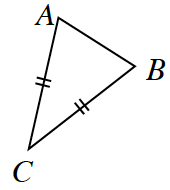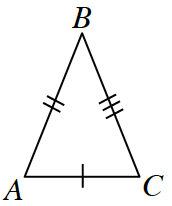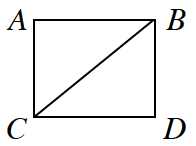### Home > GC > Chapter 1 > Lesson 1.3.2 > Problem1-110

1-110.

If no sides of a triangle have the same length, the triangle is called scalene (pronounced SCALE-een). And, as you might remember, if the triangle has two sides that are the same length, the triangle is called isosceles. Use the markings in each diagram below to decide if $ΔABC$ is isosceles or scalene. Assume the diagrams are not drawn to scale.

Sides with the same markings mean the lengths are the same.

1.Isosceles

1.Scalene

1. $ABDC$ is a squareIsosceles